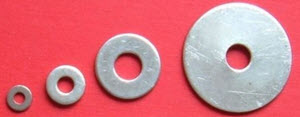# Solids of Revolution by Disks

We can have a function, like this one:And revolve it around the x-axis like this:To find its volume we can add up a series of disks:Each disk's face is a circle:The area of a circle is π times radius squared:

A = π r2

And the radius r is the value of the function at that point f(x), so:

A = π f(x)2

And the volume is found by summing all those disks using Integration:

Volume =
b
a
π f(x)2 dx

And that is our formula for Solids of Revolution by Disks

In other words, to find the volume of revolution of a function f(x): integrate pi times the square of the function.

### Example: A Cone

Take the very simple function y=x between 0 and bRotate it around the x-axis ... and we have a cone!The radius of any disk is the function f(x), which in our case is simply xWhat is its volume? Integrate pi times the square of the function x :

Volume =
b
0
π x2 dxFirst, let's have our pi outside (yum).

Seriously, it is OK to bring a constant outside the integral:

Volume = π
b
0
x2 dx

Using Integration Rules we find the integral of x2 is: x33 + C

To calculate this definite integral, we calculate the value of that function for b and for 0 and subtract, like this:

Volume = π (b33033)

= π b33

Compare that result with the more general volume of a cone:

Volume = 1 3 π r2 h

When both r=b and h=b we get:

Volume = 1 3 π b3

As an interesting exercise, why not try to work out the more general case of any value of r and h yourself?

We can also rotate about other lines, such as x = −1

### Example: Our Cone, But About x = −1

So we have this:Rotated about x = −1 it looks like this:The cone is now bigger, with its sharp end cut off (a truncated cone)

Let's draw in a sample disk so we can work out what to do:OK. Now what is the radius? It is our function y=x plus an extra 1:

y = x + 1

Then integrate pi times the square of that function:

Volume =
b
0
π (x+1)2 dx

Pi outside, and expand (x+1)2 to x2+2x+1 :

Volume = π
b
0
(x2 + 2x + 1) dx

Using Integration Rules we find the integral of x2+2x+1 is x3/3 + x2 + x + C

And going between 0 and b we get:

Volume = π (b3/3+b2+b − (03/3+02+0))

= π (b3/3+b2+b)

Now for another type of function:

### Example: The Square Function

Take y = x2 between x=0.6 and x=1.6Rotate it around the x-axis:What is its volume? Integrate pi times the square of x2:

Volume =
1.6
0.6
π (x2)2 dx

Simplify by having pi outside, and also (x2)2 = x4 :

Volume = π
1.6
0.6
x4 dx

The integral of x4 is x5/5 + C

And going between 0.6 and 1.6 we get:

Volume = π ( 1.65/5 − 0.65/5 )

6.54

Can you rotate y = x2 about x = −1 ?

## In summary:• Have pi outside
• Integrate the function squared
• Subtract the lower end from the higher end

We can also rotate about the Y axis:

### Example: The Square Function

Take y=x2, but this time using the y-axis between y=0.4 and y=1.4Rotate it around the y-axis:And now we want to integrate in the y direction!

So we want something like x = g(y) instead of y = f(x). In this case it is:

x = √(y)

Now integrate pi times the square of √(y)2 (and dx is now dy):

Volume =
1.4
0.4
π √(y)2 dy

Simplify with pi outside, and √(y)2 = y :

Volume = π
1.4
0.4
y dy

The integral of y is y2/2

And lastly, going between 0.4 and 1.4 we get:

Volume = π ( 1.42/2 − 0.42/2 )

2.83...

## Washer MethodWashers: Disks with Holes

What if we want the volume between two functions?

### Example: Volume between the functions y=x and y=x3 from x=0 to 1

These are the functions:Rotated around the x-axis:The disks are now "washers":And they have the area of an annulus:In our case R = x and r = x3

In effect this is the same as the disk method, except we subtract one disk from another.

And so our integration looks like:

Volume =
1
0
π (x)2π (x3)2 dx

Have pi outside (on both functions) and simplify (x3)2 = x6:

Volume = π
1
0
x2 − x6 dx

The integral of x2 is x3/3 and the integral of x6 is x7/7

And so, going between 0 and 1 we get:

Volume = π [ (13/3 − 17/7 ) − (0−0) ]

≈ 0.598...

So the Washer method is like the Disk method, but with the inner disk subtracted from the outer disk.